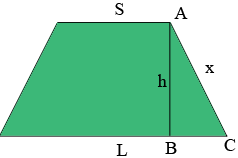SEARCH HOMEMath Central Quandaries & QueriesQuestion from Riham: Hi How can I find the height in an isosceles trapezoid if I have the measurements of all of its sides ? Thank u in advance.Hi,

Suppose the length of the longer of the parallel sides is $L$ units, the length of the shorter of the parallel sides is $S$ units and the lengths of the other two sides is $x$ units as in the diagram below.The length of the line segment BC is $\large \frac{L-S}{2},$ can you see why? Thus for the right triangle $ABC$ you know the lengths of two of its sides and Pythagoras Theorem will give you $h,$ the length of the third side.

PennyMath Central is supported by the University of Regina and The Pacific Institute for the Mathematical Sciences.